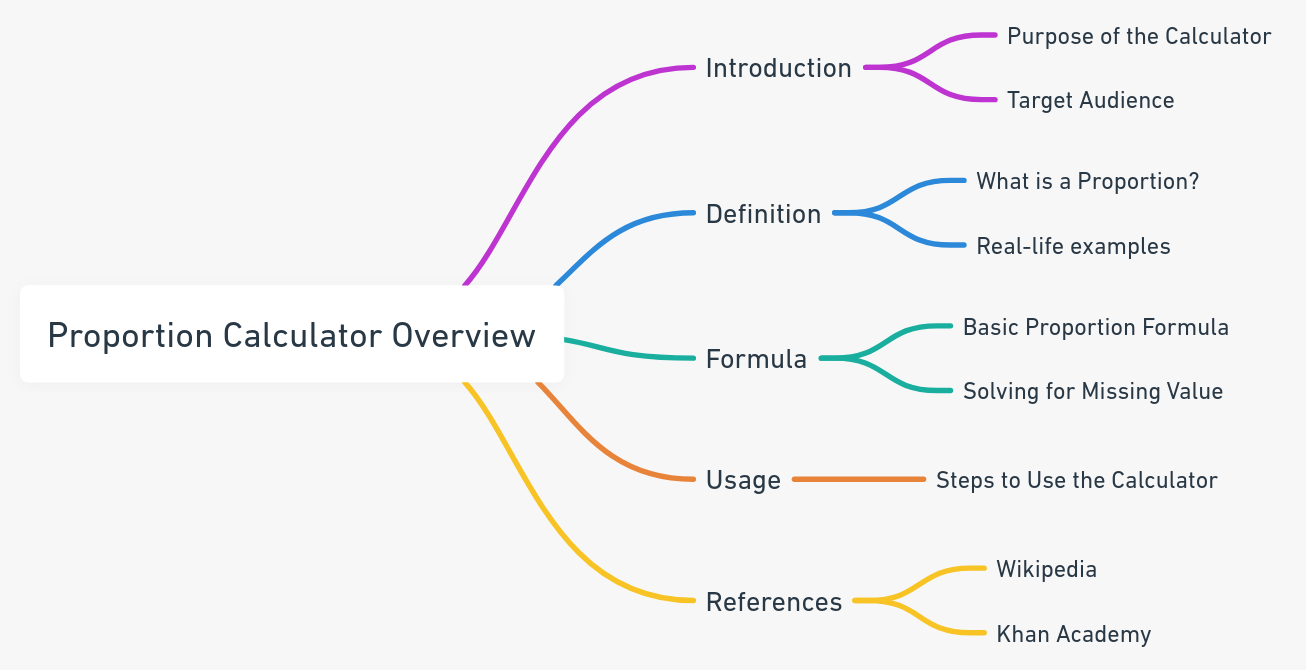Even The mini Tools Can Empower People to Do Great Things.

# Proportion Calculator: Step-by-Step Solutions in Seconds

Proportion Calculator
Enter 3 numbers below and leave one field blank to solve for that value.
 =

Embed Proportion Calculator WidgetThe Proportion Calculator is used to solve proportion problems and find the missing value in a proportion (Step by Step). Whether you're a student, teacher, or professional, understanding and calculating proportions is now at your fingertips.

## What is a Proportion?

A proportion represents the relationship between two quantities, signifying that two ratios are equivalent. For instance, the fraction 1/3 is equivalent to 2/6, showcasing the fundamental concept of proportions.

## Understanding the Formula of Proportion:

The essence of a proportion lies in its formula, which allows us to equate two ratios and solve for an unknown value. The basic formula for proportion is represented as:

$\frac{a}{b} = \frac{c}{d}$

Where:

1. (a) and (b) are values from the first ratio.
2. (c) and (d) are values from the second ratio.

To solve for a missing value in a proportion, you can cross-multiply and then solve for the unknown variable. For instance, if you know (a), (b), and (c) but want to find (d), you can rearrange the formula:

$d = \frac{b \times c}{a}$

Our Proportion Calculator not only gives you the result but also provides a step-by-step breakdown using this formula, ensuring you understand the calculation process thoroughly.

## How to Use the Proportion Calculator?

To solve a proportion using our calculator:

1. Enter 3 known values.
2. Leave one field blank.
3. Click the Calculate button to get your result.

## Reference

Reference this content, page, or tool as:

"Proportion Calculator" at https://miniwebtool.com/proportion-calculator/ from miniwebtool, https://miniwebtool.com/

by miniwebtool team. Updated: Oct 07, 2023

## Related Miniwebtools:

Fraction Calculator